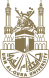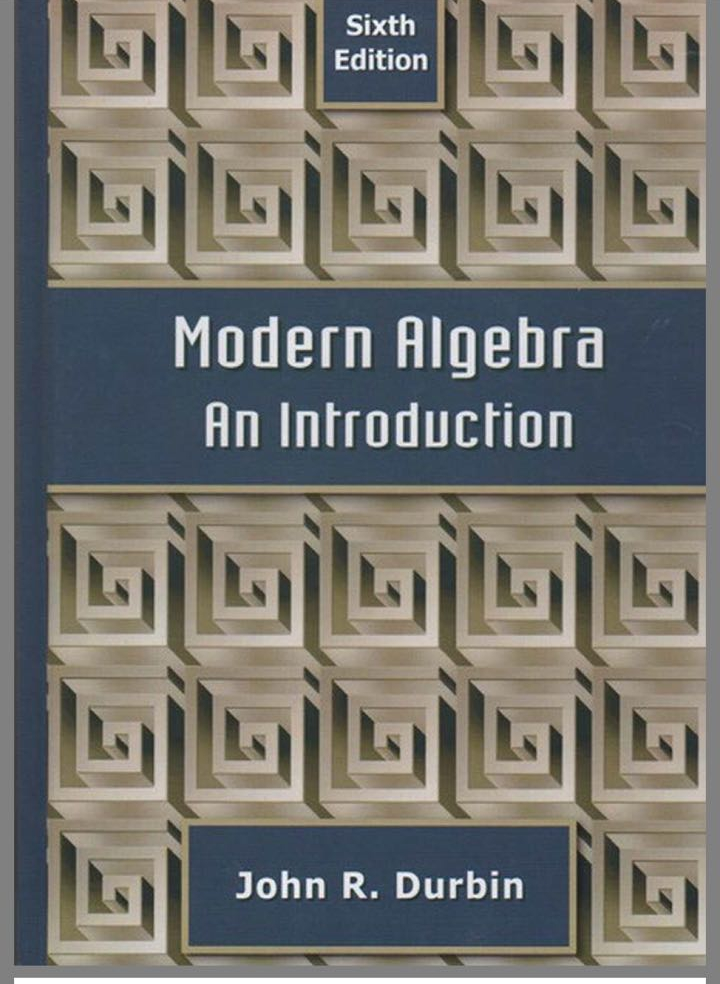Umm Al-Qura UniversityIntroduction to Group Theory 3-4043403

- 2018/09/09

Introduction to Group Theory Math. 3-4043403

1. Course Content:
 List of Topics No. of Weeks Introduction and examples: Definition of a group, subgroups, examples 1-2 Cyclic groups,  generating sets and Caley Diagrams 3-4 Permutations, groups of symmetries (triangle, rectangle, square),   Co-sets, and Direct Products of groups. 5-6 Homomorphisms between groups normal subgroups  and factor groups, automorphisms of groups 7-8 Group Action on a set (Orbit Stabilizer Theorem), fixed points, p-groups, examples 9-11 conjugacy classes, class equations 12-13 Sylow theorems of finite groups 14-15

2- Suggested bookBook content